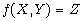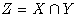6.4 Functor Definition

We will explain the fuctor definition next. For example, let us think about a 2 variable fuctor. Let us assume that we want to make a new set Z from the set (set)X,Y. We will explain what to do at this point.

X /\ Y → Z
set  set  set

definition
let X , Y be set;
func X /\ Y　->　set
means
:N: x in it iff
x in X　&　x in Y;
existence ......;
uniqueness
proof
end;
end;

This case also allows for it to be written in the operation form instead of the definition of a function like. Let us assume that we want to define such a symbol as. To make a new set Z from sets X and Y is truly this functor. With let X,Y be set;, we have sets X, Y ready. In addition, func X
/\ Y -> set indicates X /\ Y as a set. The next line after means is the meaning of that functor. We will then write the meaning of the functor by placing a label like before. In this meaning, X /\ Y is indicated as it.
In addition, in the demonstration section (contents of the meaning) , we have to demonstrate the existence and uniqueness. In other words, we have to express its existence and uniqueness (the existence is only one).
For the demonstration for uniqueness, we only have to state the following.
If we take A1 and A2 as it:

(x in A1 ⇔ x in X & x in Y)
and (x in A2 ⇔ x in X & x in Y)
⇒　　A1 = A2

If we write this, it will be as below:

uniqueness
proof
let A1,A2　be set such that
A1: x in A1 iff x in X　&　x in Y
and
A2: x in A2　iff x in X　&　x in Y;
now let y;
y in A1 iff y in X　&　y in Y by A1;
hence y in A1 iff y in A2　by A2;
end:
hence A1=A2 by TARSKI:2;

In short, when we bring in the two sets A1 and A2 that satisfy this condition, by demonstrating the equality of these two sets, the uniqueness is in fact demonstrated.
In this demonstration of uniqueness, because it is such that in the third line, one can see that after it the two sentences label A1 and label A2 are connected with and. It is stated as now let y. Let us assume that this y is reserved as set. In addition, by label A1 we can state as y in A1 iff y in X & y in Y, and therefore, by label A2, y in A1 iff y in A2 can be stated. In addition, it means that enough necessary conditions for A1=A2 are demonstrated by TARSKI:2 stating that y in A1 and y in A2 are equally corresponding with the optional y; therefore, A1=A2 are demonstrated.
We also have to demonstrate the existence in the same way; however, we have to state that such an it exists. That is, let us demonstrate for the following.

ex A being set st for x
holds x in A1 iff x in X　&　x in Y